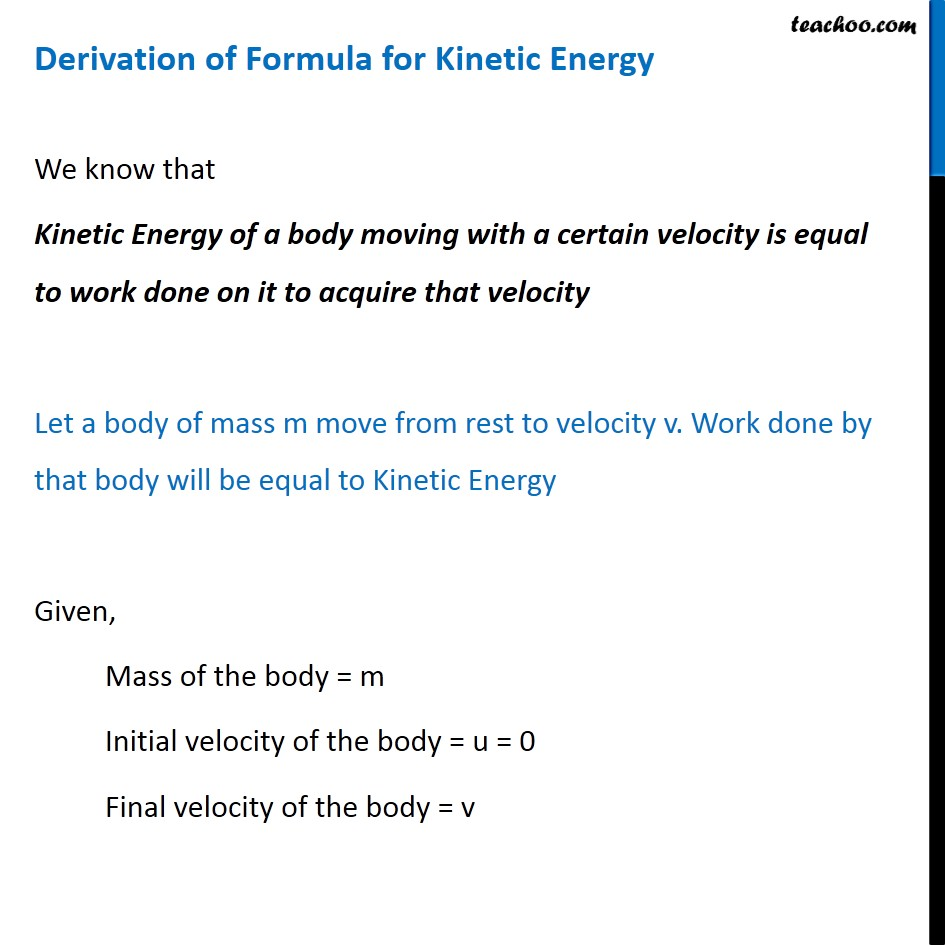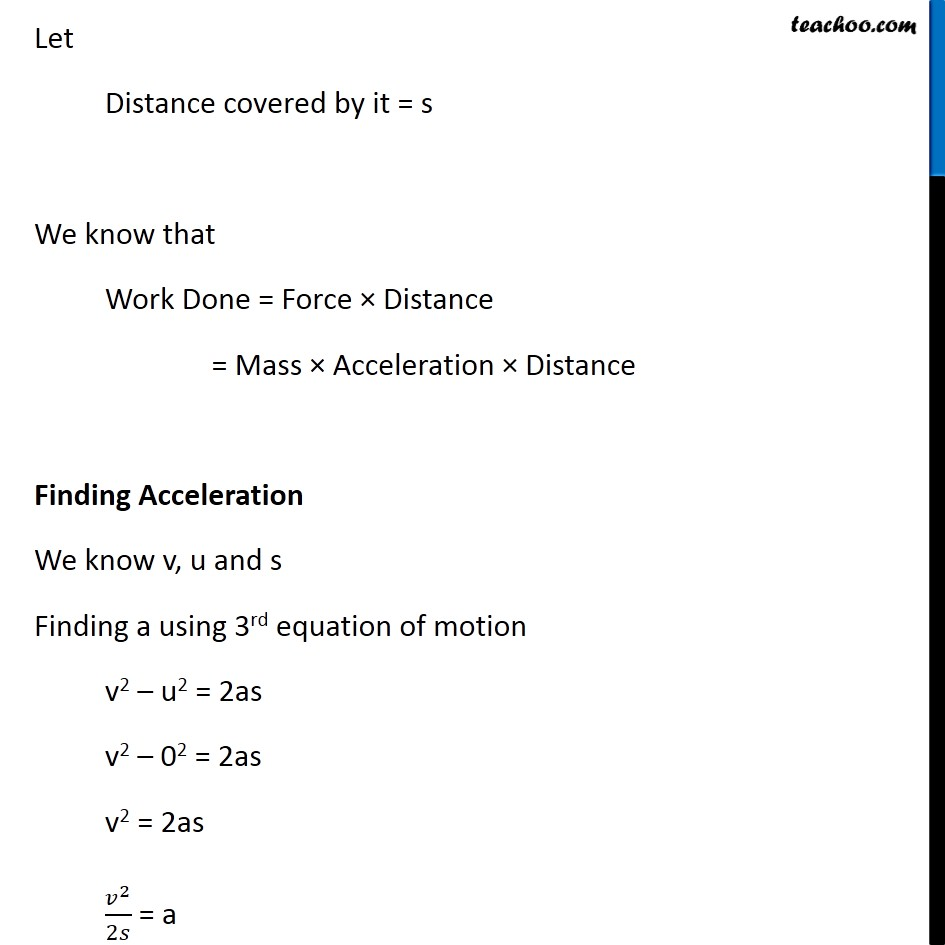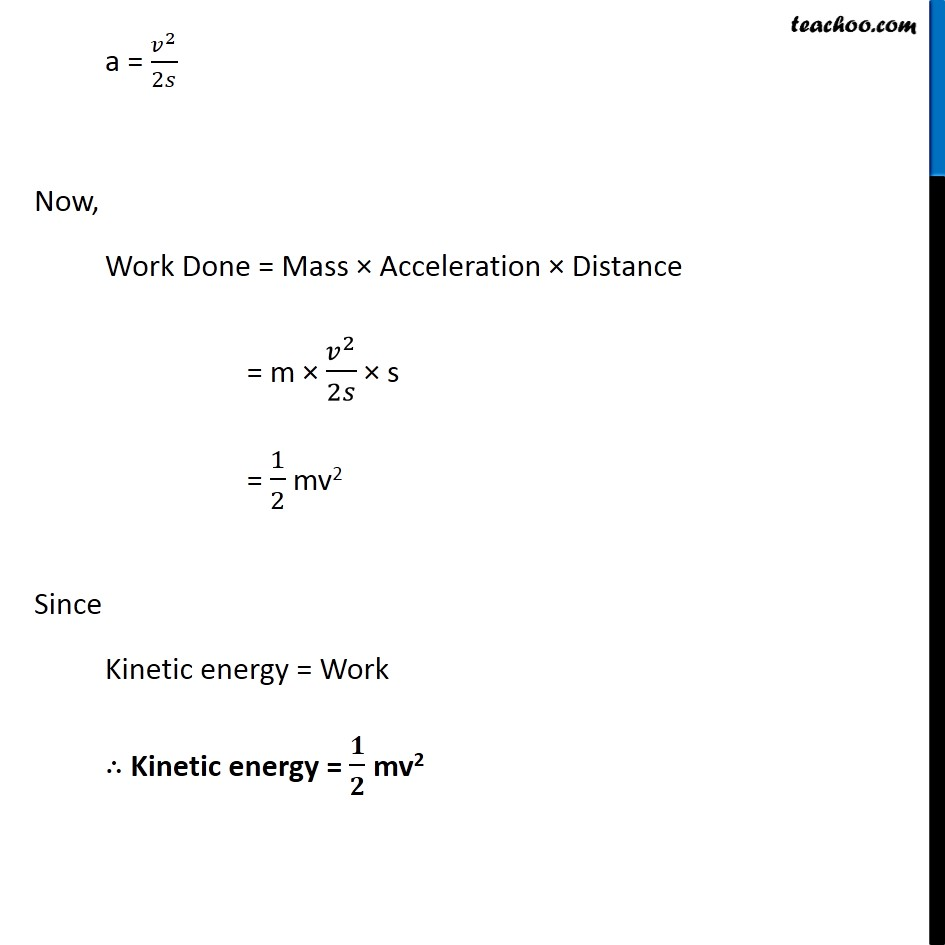Concepts

Class 9
Chapter 11 Class 9 - Work and Energy

The energy of a body due to its motion is called kinetic enerrgy

Every moving body posseses kinetic energy

Example 1

A ball kept at ground does not have kinetic enrgy

But a ball thrown at batsmen has kinetic energy

Example 2

A bullet kept in a gun does not have kietic energy

But a bullet fired from gun has kinetic energy## How to measure kinetic energy of a moving body

It is the amount of work a body can do before coming to rest

Example

If we kick a football, it travels some distance before coming to a rest

Work done by football in travelling a distance before coming to rest is kinetic energy

In this case, when football is kicked,it has certain initial velocity u

When ball stops, its final velocity v becomes 0

## Formula for Calculating Kinetic Energy

The formula for Kinetic energy is

Kinetic Energy = 1/2 × Mass × Velocity^2

Kinetic Energy = 1/2 × mv^2

## How is this formula derived

To derive this formula, we use the relation between Kinetic Energy and Work Done

Kinetic Energy of a body moving with a certain velocity is equal to work done on it to acquire that velocity

Let's see its derivationNote: While doing questions, we use

Change in Kinetic Energy = Work Done

## Questions

Q 1 Page 152 - What is the kinetic energy of an object?

Q 2 Page 152 - Write an expression for the kinetic energy of an object

Q 3 Page 152 - The kinetic energy of an object of mass, m moving with a velocity of 5 m s–1 is 25 J. What will be its kinetic energy when its velocity is doubled? What will be its kinetic energy when its velocity is increased three times?

Example 11.3 - An object of mass 15 kg is moving with a uniform velocity of 4 m s–1. What is the kinetic energy possessed by the object?

Example 11.4 - What is the work to be done to increase the velocity of a car from 30 km h–1 to 60 km h–1 if the mass of the car is 1500 kg?

NCERT Question 4 - Certain force acting on a 20 kg mass changes its velocity from 5 m s–1 to 2 m s–1. Calculate the work done by the force.

NCERT Question 16 - An object of mass, m is moving with a constant velocity, v. How much work should be done on the object in order to bring the object to rest?

NCERT Question 17 - Calculate the work required to be done to stop a car of 1500 kg moving at a velocity of 60 km/h?

Learn in your speed, with individual attention - Teachoo Maths 1-on-1 Class

### Transcript

Derivation of Formula for Kinetic Energy We know that Kinetic Energy of a body moving with a certain velocity is equal to work done on it to acquire that velocity Let a body of mass m move from rest to velocity v. Work done by that body will be equal to Kinetic Energy Given, Mass of the body = m Initial velocity of the body = u = 0 Final velocity of the body = v Let Distance covered by it = s We know that Work Done = Force × Distance = Mass × Acceleration × Distance Finding Acceleration We know v, u and s Finding a using 3rd equation of motion v2 – u2 = 2as v2 – 02 = 2as v2 = 2as 𝑣^2/2𝑠 = a a = 𝑣^2/2𝑠 Now, Work Done = Mass × Acceleration × Distance = m × 𝑣^2/2𝑠 × s = 1/2 mv2 Since Kinetic energy = Work ∴ Kinetic energy = 𝟏/𝟐 mv2